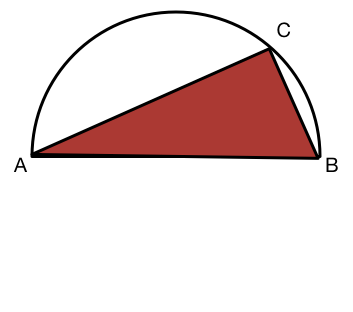# In a semicircle$AB$ is the diameter of a semi-circle.
$C$ is a point on the circumference.
What is the measure of angle $ACB?$

×